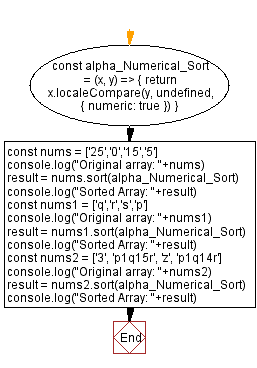# JavaScript Sorting Algorithm: Alpha Numerical Sort

## JavaScript Sorting Algorithm: Exercise-26 with Solution

From Wikipedia,
In computing, natural sort order (or natural sorting) is the ordering of strings in alphabetical order, except that multi-digit numbers are treated atomically, i.e., as if they were a single character. Natural sort order has been promoted as being more human-friendly ("natural") than machine-oriented, pure alphabetical sort order. For example, in alphabetical sorting, "z11" would be sorted before "z2" because the "1" in the first string is sorted as smaller than "2", while in natural sorting "z2" is sorted before "z11" because "2" is treated as smaller than "11".

Alphabetical sorting:

• z11
• z2

Natural sorting:

• z2
• z11

Write a JavaScript program to sort a list of elements using the Alpha Numerical sorting algorithm.

Sample Data: Original array: [‘25’,’0’,’15’,’5’]
Sorted Array: [‘0’,’5’,’15’,’25’]
Original array: [‘q’,’r’,’s’,’p’]
Sorted Array: [‘p’,’q’,’r’,’s’]
Original array: [‘3’, ‘p1q15r’, ‘z’, ‘p1q14r’]
Sorted Array: [‘3’, ‘p1q14r’, ‘p1q15r’, ‘z’]

Sample Solution:

JavaScript Code:

``````const alpha_Numerical_Sort = (x, y) => {
return x.localeCompare(y, undefined, { numeric: true })
}
const nums = ['25','0','15','5']
console.log("Original array: "+nums)
result = nums.sort(alpha_Numerical_Sort)
console.log("Sorted Array: "+result)
const nums1 = ['q','r','s','p']
console.log("Original array: "+nums1)
result = nums1.sort(alpha_Numerical_Sort)
console.log("Sorted Array: "+result)

const nums2 = ['3', 'p1q15r', 'z', 'p1q14r']
console.log("Original array: "+nums2)
result = nums2.sort(alpha_Numerical_Sort)
console.log("Sorted Array: "+result)
```
```

From mozilla.org -
The localeCompare() method returns a number indicating whether a reference string comes before, or after, or is the same as the given string in sort order. In implementations with Intl.Collator API support, this method simply calls Intl.Collator.

Sample Output:

```"Original array: 25,0,15,5"
"Sorted Array: 0,5,15,25"
"Original array: q,r,s,p"
"Sorted Array: p,q,r,s"
"Original array: 3,p1q15r,z,p1q14r"
"Sorted Array: 3,p1q14r,p1q15r,z"
```

Flowchart:Live Demo:

See the Pen searching-and-sorting-algorithm-exercise-26 by w3resource (@w3resource) on CodePen.

Improve this sample solution and post your code through Disqus

Previous: Timsort sorting algorithm.
Next: Binary Insertion sort.

What is the difficulty level of this exercise?

Test your Programming skills with w3resource's quiz.

﻿